HomeBlog

# How to Distinguish Resistor Color Codes? (Axial Resistors)

Introduction

What is a resistor?

The resistor is a passive electrical component to create resistance when electric current flowing. We can find it in all electrical networks and electronic circuits. The resistance is measured in ohms. An ohm is the resistance that occurs when a current of current passes through a resistor with a one volt drop across its terminals. Commonly used color band resistor can be divided into four-band and five-band, in practice, usually four-band. The front two bands of the four-band resistor is the number, the third band is the number of times resistance, the last band is the error; the front three bands of the five-band resistor is the number, the fourth band is the number of times the resistance, and the last one is the error. The error-band color is usually gold, silver and brown. The error of gold is 5%, the error of silver is 10%, the error of brown is 1%, the error of colorless is 20%. In addition, green will represent the error, its value is 0.5%. Precision resistors are usually used in military, aerospace, etc. The color-band resistor in the earliest days was to help people to distinguish the resistance.

Catalog

 Article Core: Resistor Color Codes Application: Cylindrical Resistors Recognition Sequence: Three Tips Recognition Method : Two Parts Classification: Three-band-code ResistorFour-band-code ResistorFive-band-code ResistorSix-band-code Resistor

How to Read a Resistor

Resistors are used and manufactured by thousands of organizations and people all over the world. So we should know  what it is and its functions well, therefore, it has many classifications based on different standards, we must tell it one by one, this article is mainly discuss the color-band resistors.

To identify the value of color-band resistors, a resistor color code is used. This color code consists of several colorful bands. Surface mount resistors are identified by a numerical resistor code. The nominal values of resistors are also standardized. Several ranges of preferred resistor values are available. Another important aspect of resistor standardization is the use of standardized resistor symbols. The IEC standard symbol of a fixed value resistor is shown.

Color-band resistor is the most commonly used electronic component in electronic circuits. The color-ring resistor is used to distinguish the resistance value of the resistor by coating the color band with different colors on the ordinary resistor package. Ensure that resistance can be clearly read in any direction when installing the resistors. The basic units of the color ring resistor are: ohms (Ω), KΩ, MΩ, 1MΩ= 1000KΩ = 1000000Ω.

An electronic color code is used to indicate the values or ratings of electronic components, usually for resistors, but also for capacitors, inductors, diodes and others. A separate code, the 25-pair color code, is used to identify wires in some telecommunications cables. Different codes are used for wire leads on devices such as transformers or in building wiring.

A carbon-composition resistor can have 4 to 6 bands. A 5-band resistor is more precise compared to a 4-band type because of the inclusion of a third significant digit. A 6-band resistor is like a 5-band resistor but includes a temperature coefficient band (the 6th band).

 4-band 5-band 6-band 1st band 1st significant digit 1st significant digit 1st significant digit 2nd band 2nd significant digit 2nd significant digit 2nd significant digit 3rd band multiplier 3rd significant digit 3rd significant digit 4th band tolerance multiplier multiplier 5th band N/A tolerance tolerance 6th band N/A N/A Temperaturecoefficient

Resistor Color Codes mean that the resistance is represented by four or five color rings or six color rings above the resistor. The color information representing the resistance value can be read at any time at a time. So resistors with color codes is the most widely used in various electronic devices. No matter how it is installed, the repairman can easily read its resistance value, which is easy to detect and replace.

Resistor Color Code Values

Each color represents a number if it's located from the 1st to 2nd band for a 4-band type or 1st to 3rd band for a 5-band and 6-band type.If the color is found on the 3rd band for a 4-band type or the 4th band for a 5-band and 6-band type, then it's a multiplier.The 6th band for a 6-band type resistor is the temperature coefficient. This indicates how much the actual resistance value of the resistor changes when the temperature changes.Application

Color band marks are mainly used on cylindrical resistors, such as carbon film resistor, metal oxide film resistor, fuse resistor, wire winding resistor.

Recognition Sequence

However, it has been found in practice that some color-band resistors are not arranged in a very clear order and are often easy to misread. In recognition, the following techniques can be used to judge:

Tip 1: Find the color band of the mark error first, and then arrange the color band order. The most commonly used colors to indicate resistance errors are: gold, silver, brown. In addition, gold and silver rings, which are rarely used as the first ring of a resistor color ring, so long as there is a gold band or a silver band on the resistor, this can basically be recognized as the last band of color-band resistor.

Tip 2: Brown band is usually identified as an error mark. Brown band is often used as error band or as effective number band, and it often appears in the first band and the last band synchronously, which makes it difficult to identify who is the first band. In practice, it can be judged by the gap between the color bands: for example, for a five-band-code resistor, the gap between the fifth and fourth band is wider than that between the first and second band. Based on this, the arrangement order of color bands can be determined.

Tip 3: In the case that the color band order can not be determined by the color band spacing alone, it can also be judged by the production sequence value of the resistor. For example, there is a color band reading order of resistance: brown, black, black, yellow, brown, its value is: 100 × 10000mΩ, the error is 1%, belonging to the normal resistance series value, if read in reverse order: brown, yellow, black, black, brown, its value is 140 × 1Ω = 140Ω, the error is 1%. Obviously, the resistance values read out in the latter order is wrong according to production standard of resistors, so the order of the latter color loops is incorrect.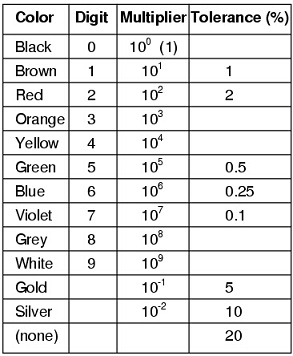Recognition Method

In the early days, when the surface of the resistor was not sufficient to display all resistor values by numbers, the resistance, tolerance, and specification of the resistor were expressed by the color band marking method. There are two main parts.

Part one: a group near the front end of the resistor is used to indicate the resistance value.

The resistance value of a two significant numbers, represented by the first three color rings, such as 39Ω, 39KΩ, 39MΩ.

The resistance value of the three significant resistance numbers is represented by the first four color rings, such as: 69.8Ω, 698Ω, 69.8KΩ, which is generally used to express the precision resistor.

Part two: a color band near the rear end of the resistor is used to represent tolerance accuracy.

Each color ring in the first part is equidistant and easily distinguished from the second color band.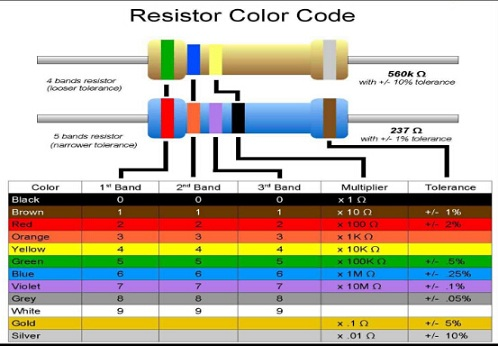Four-band-code Resistor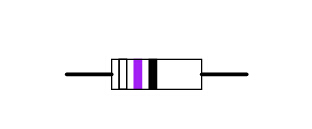The four-color band resistor is identified as follows: the first and second band represent the resistance of the two-digit significant number; the third band represents the multiplier; and the fourth band represents the error.

Examplebrow, red, red, gold

Its resistance value is 12 × 10 ^ 2= 1.2kΩ, the error is ±5%.

The error also represents, it fluctuates around the standard value of 1200, about 5% × 1200, this resistance is acceptable, that is, the resistance is good between 1140~1260.

The first and second ring represent the first two digits of the four-color resistor respectively; the third ring represents the multiplier; the fourth band represents the error. The key to fast recognition is to determine the resistance value within a certain order of magnitude according to the color of the third band, for example, when the number is a few Ks or dozens of K, and then connect with the numbers in the first and second band, so that the final resistance can be read out quickly.

For the four-band-code resistor, the method of calculating the resistance value is as follows:

Resistance= (first color-ring value * 10+second color+ring value) * the multiplier represented by the 3rd color band.

Five-band-code Resistor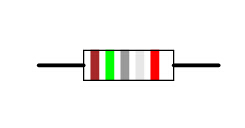The recognition of five-band-code resistors: the first, second and third bands represent the resistance of the three-digit significant number respectively; the fourth band represents the multiplier; the fifth ring represents the error. If the fifth color band is black, it is generally used as a wire wound resistor, and the fifth color band, if white, is generally used as a fuse resistor. If the resistor has only a black color band in the middle, it represents zero ohmic resistance.

Example: red, red, black, brown, gold

Its resistance is 220 × 10 ^ 1 / 2.2KΩ, the error is ±5%.

The first color band is hundreds digit, the second color band is tens digit number;

The third color band is the single digit, the fourth color band is the color power;

The fifth color band is the error rate.

For the five-band-code resistor, the method of calculating the resistance is as follows:

Resistance= (first color-band value * 100+second color band value * 10+third color-band value) * the multiplier of the fourth color band.

Six-band-code Resistor

The identification of this resistor is the same as the above mentioned resistors, but the sixth color ring represents the temperature coefficient of the resistor.

Examples

Example 1: when the four color rings are yellow, orange, red and gold, because the third band is red, the resistance range is single digit kΩ. According to the number "4" and "3" of yellow and orange respectively, the reading number is 4.3 kΩ. The fourth band is gold representing the error of 5%.

Example 2: when the four color bands are brown, black, orange and gold in turn, because the third band is orange and the second ring is black, the resistive value should be tens of kΩ, the number "1" of brown is substituted, and the reading number is 10 kΩ. The fourth band is gold, and the error is 5%. In some indistinguishable cases, you can also compare the colors of the two ends, because the first color, will not be gold, silver, or black. If these three colors are close to the edge, they need to be calculated backwards.

There are two ways to identify the colorful resistor. One is to label the color band with 4 color rings, the other is to label the color bandwith 5 color bands. The difference between the two is that the first two bits of the four-color band represent the effective number of the resistor, but the first three bits of the five-color band resistor represent the effective numbers, and the last but one represents the multiplier of the effective number of the resistor. The last bit represents the error of the resistor.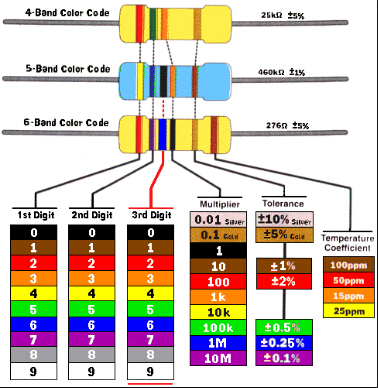You May Also Like

Some Basic Knowledge about Resistor

Product Recommendation

KY83-RL7520WT-R020-F

KY0-MP9100-0.10-1%

MPMT20018001AT1HOW TO BUY ELECTRONIC COMPONENTS
How to buy
Search
Inquiry
Order
Track

Delivery
FedEx
UPS
DHL
TNT

Payment Terms
By PayPal
By Credit Card
By Wire Transfer
By Western Union

After-sales Service
Quality Control
Guarantee
Return & Replacement

About us
Company Profile
Our History
Corporate Culture
Contact us
Join us
© 2008-2018 kynix.com all rights reserved.
Tel:00852-81928838    Email:info@kynix.com